Originally unsharp masking process was invented in photograph. A negative and its positive film are hybrid and produce a detail image. This process is a multiplication of two films. However, most software use a subtractive unsharp masking process. So do I. Why? My reason was that the subtractive one was just very similar to the photograph one that I did with a film taken by my Schmidt camera.
Someone asked me what is the difference between subtractive and dividing one. Then, I compared these two processes. Making a unfocus image, I used the average unfocus. The average one is similar to the photograph one because an optical defucus is average. Some people use the gaussian unfocus image, but these are not so different. I will mention about this later. In the dividing unsharp masking process, we have to avoid zero intensity because it cannot be divided by zero. I put a bias 100 counts into the unsharp mask for the dividing one.
Let's see the comparison first.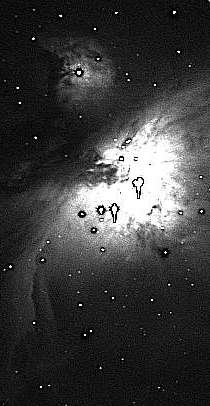Left : Deviding unsharp makins , Right : Subtractive unsharp masking

From these results, we know the dividing unsharp masking process is much better than subtractive one.
Why so ? The subtractive one produces the difference of intensities in small regions. The difference in a faint part is small, and the difference in a bright part is big. Therefore, 8 bit image can't cover a whole range. On the other hand, the dividing one produces the ratio of intensities in small regions. The ratio doesn't matter how it is bright or faint, so the dividing unsharp mask process shows us a whole image from brighter parts to fainter parts.
Let me give you an example of a galaxy. Let a center intensity of this galaxy 100 counts, and an arm 1 count. Making unsharp mask, let the center intensity of the mask 50 and the arm intensity of the mask 0.5.
Substractive unsharp masking process produces 100-50=50 in the center and 1.0-0.5=0.5 in the arm. Dividing one produces 100/50=2.0 in the center and 1.0/0.5=2.0 in the arm. We know the latter one covers from the center to the arm in same level.

The dividing process can be called optical unsharp mask because an original image divided by unsharp mask corresponds to an image created by optical way. The equation of this process is y = x/( <x> + b ). " b " is a bias to avoid dividing by zero. In optics, clear glass has 1.0 multiplication, but not 0.0, so " b " has to be more than <x>, average of several pixels around x. According to Okano Kunihiko's Digital Development, he uses the hyperboric transfer to compress a wide dynamic range image. The equation is y = x/( x+ a ). To make it edge enhanced, he modifies this equation to y = x / ( <x> + a ). This is definitely same to the optical unsharp mask process.

Sabtractive unsharp mask process is a kind of convolution process.
I was thinking how we interrupt the subtractive unsharp mask. It is different from original one, but looks like the one. Let's think about the process.
2nd, the original image is subtracted by the mask
The mask is created by a convolution with gaussian PSF. The original image is a convolution with PSF of a pinpoint. Subtractive mask means a convolution with a negative gaussian PSF. Therefore, all process is done by convolutions. See the diagram.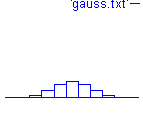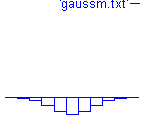Left : a gaussian PSF , right : a negative gaussian PSF

Adding two convolutions is equal to a convolution with a PSF added two PSFs. Thus, we can add the negative PSF into the PSF of a pinpoint. I call this a subtractive unsharp mask PSF. See below a equation and diagram.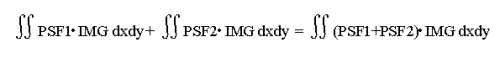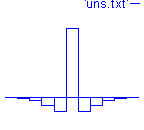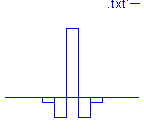Left : a pinpoint PSF , middle : a subtractive unsharp PSF , right : a typical edge ehance PSF

The mddle one is nothing more than a PSF for edge enhance. We have been misunderstanding about unsharp mask process on software. The subtractive one is a deconvolution, maybe called a convolution for edge enhance, and the dividing one is absolutely original unsharp mask. See below a comparison. The left one is a deconvolution with the subtractive unsharp mask PSF, and the other one is a subtractive unsharp mask. They are same because of upper explanation. This deconvolution can be called one of the high-pass filters, but not unsharp mask.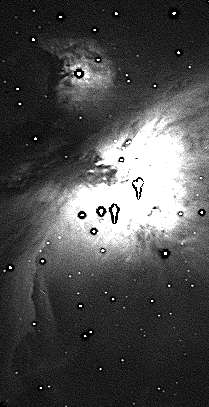Left : a convolution with PSF of a subtractive unsharp mask , right : a subtractive unsharp mask

How to make the unsharp mask?

There are some ways to blur the original image. The disk average blur is same to the optical mask, the gaussian blur seems to be the best one, and the square average blur is easiest in the computer. I made these three blurs with 5 pixels squre range. Let's see the results.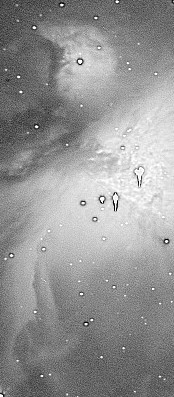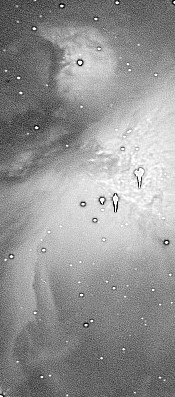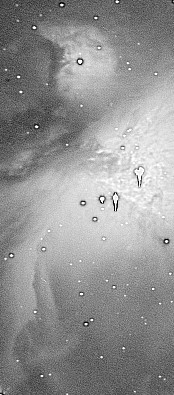There is no difference. I say that it doesn't matter how it blurs in 5x5 area.
I made an interesting mask that is blurred by a vertical rectangle, 1 by 9 pixels. The mask is blur only in vertical, so the vertical resolution is improved only. The horizontal resolution is still low.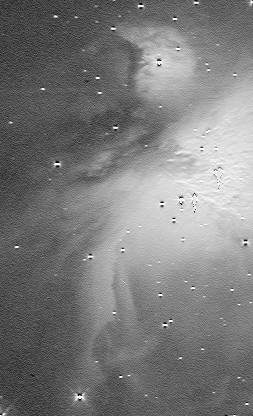Good example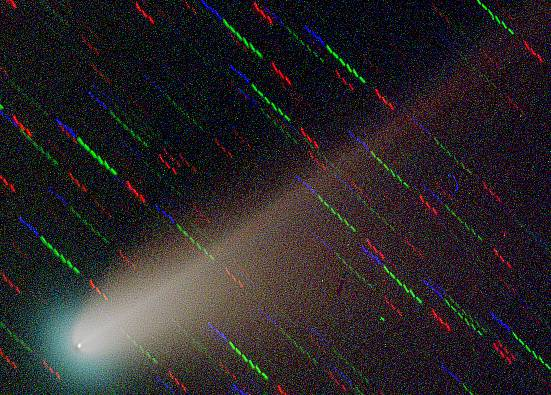Comet LINEAR S4 in July 21st, 2000

If you would like to see normal processed this comet images, click here.

12,2,2000

Astronomy

TOP

mail